All Plane-filling Curves (using edge-replacement)

 The First Nine Families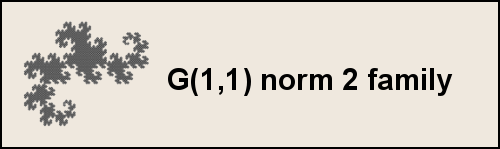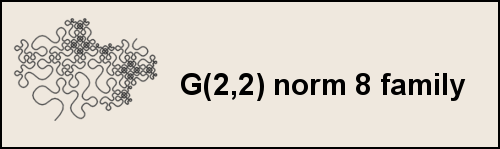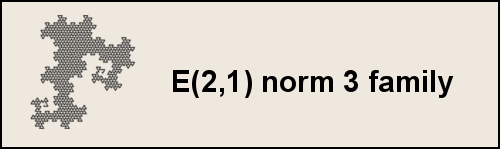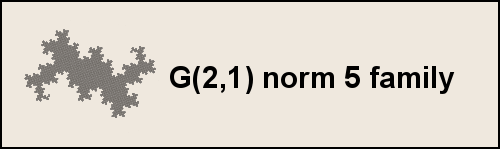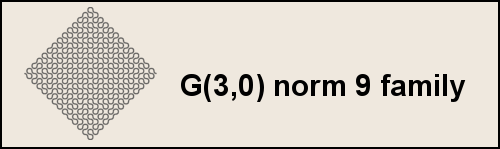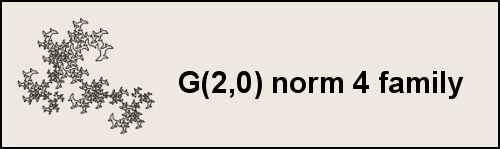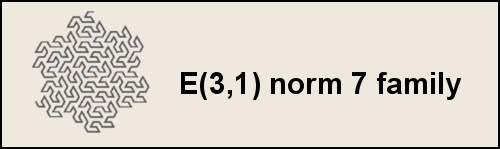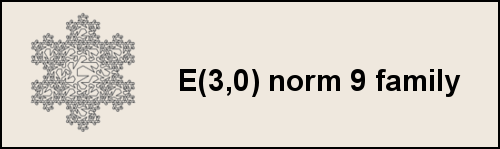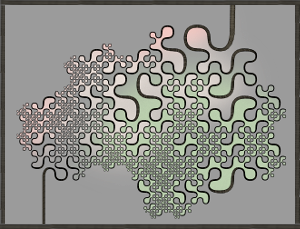The comprehensive set I have designed a comprehensive scheme for categorizing every possible plane-filling curve (2D space-filling curve) using edge-replacement (Koch construction). The technique is explained in the book, Brain-filling Curves. This method is used to both classify and generate plane-filling curves, including the familiar Original Peano curve, the Dragon curve, the Gosper curve, the Snowflake sweep, and several others. These classic curves were explained within a general framework in Benoit Mandelbrot's book, The Fractal Geometry of Nature. My system picks up where Mandelbrot left off, and introduces hundreds of new curves - with a method for finding many more.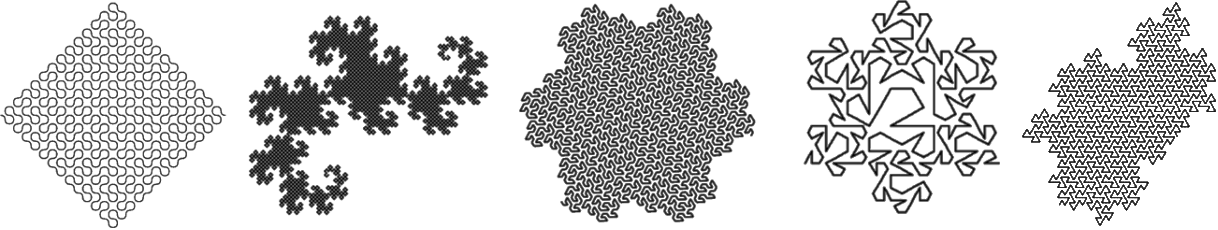Some plane-filling curves can be generated using other techniques. Another commonly used technique is 'node replacement' which is used to create the Hilbert curve, and many others. Both node-replacement and edge-replacement curves can be generated using L-systems. Also, both are related to recursive tiling. What curves qualify for this collection? All plane-filling curves using edge-replacement (Koch-construction) are included in this list. Each curve has a fractal dimension of exactly 2. It can touch itself, but it can never cross itself or overlap with itself. Not all plane-filling curves are topologically equivalent to a disc: some curves have holes or complex boundaries. However, all of them have regions that are infinitely filled - and this suffices to include them in this list. Some curves may have uneven density - and yet they are still plane-filling. While this collection does not include curves that self-overlap, a few classic self-overlapping curves are included (e.g., Cesaro's Sweep and the Peano Sweep). Relation to Gaussian and Eisenstein integers Each family f of plane-filling curves corresponds to a Gaussian integer (square lattice) or an Eisenstein integer (triangular lattice). A fractal generator can be seen as the visual expression of an ordered set of integers - each of which corresponds to one segment of the generator. The sum of these integers is the family number f. Generators of plane-filling curves can only contain segments whose associated complex number is an integer root of the family number f. Another way of saying this is that the sum of the squared lengths (the norms) of each segment must equal f. For instance, the Eisenstein-9 family is represented with generators that can have segments with norms 1 and 3. In contrast: the Eisenstein family 7 is a prime number, and so it can only have segments of length 1.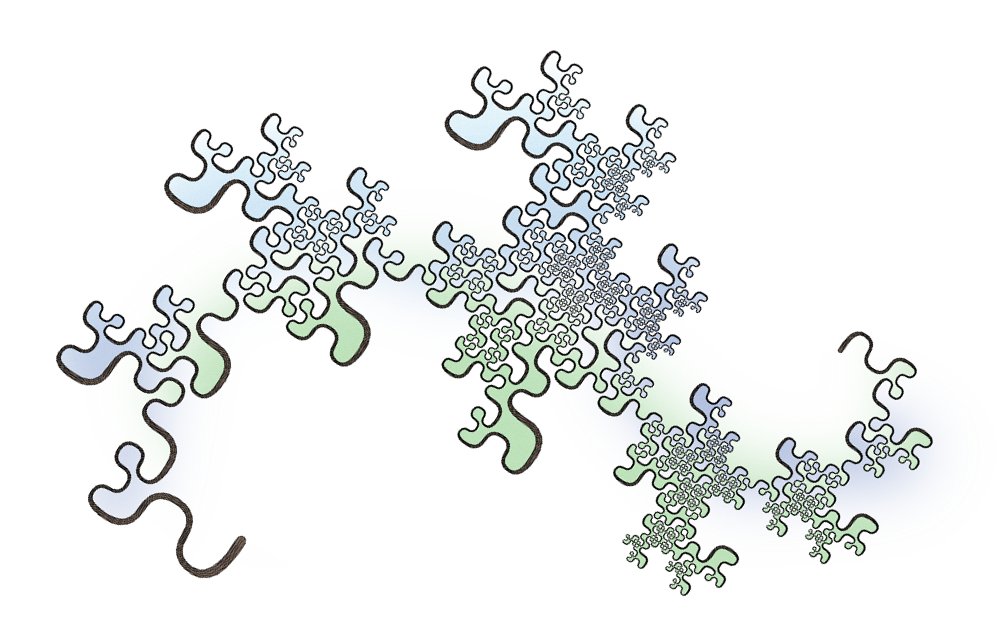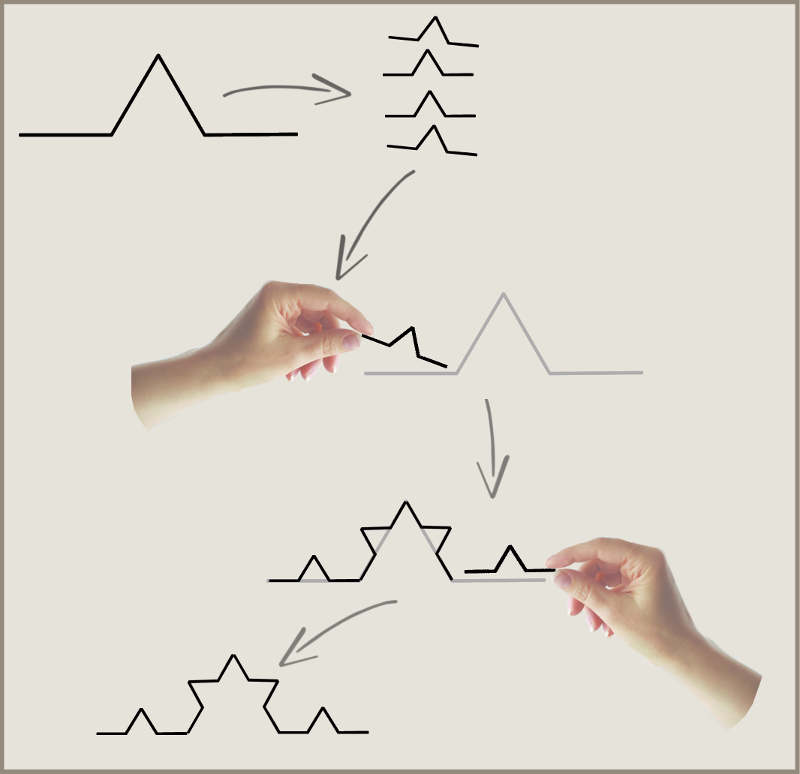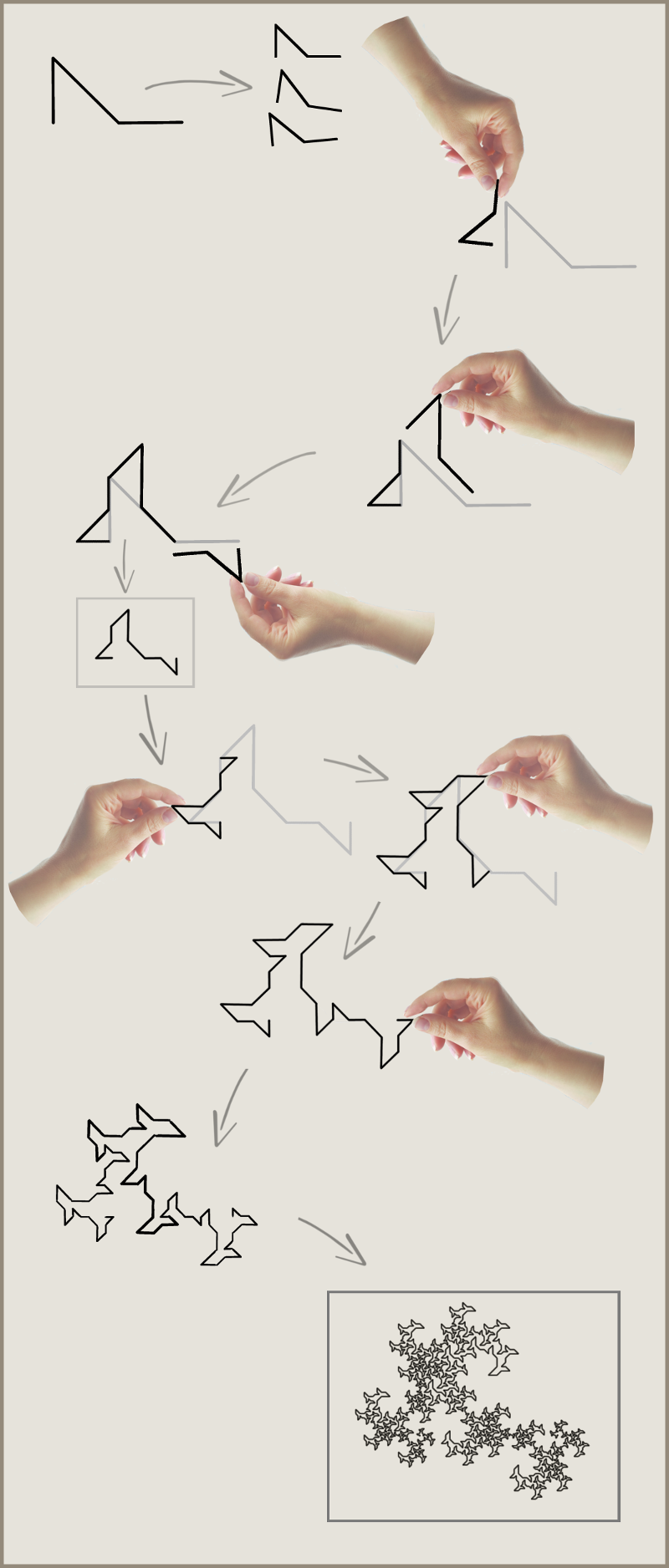The First Nine Families